# Cross-country race

In the charity cross-country race of three-member relays, each team had to run a total of 36 km. Adam, Boris and Ctirad gradually ran for team A. Boris, and Ctirad ran a distance three times longer than Adam. Ctirad ran a distance of 5 km longer than Boris.

Correct result:

a =  9
b =  11
c =  16

#### Solution:

a+b+c=36
b+c = 3a
c = 5 + b

a+b+c=36
b+c = 3•a
c = 5 + b

a+b+c = 36
3a-b-c = 0
b-c = -5

a = 9
b = 11
c = 16

Our linear equations calculator calculates it.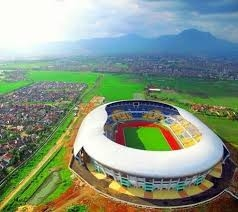We would be pleased if you find an error in the word problem, spelling mistakes, or inaccuracies and send it to us. Thank you!Tips to related online calculators
Do you have a system of equations and looking for calculator system of linear equations?
Do you want to convert length units?

## Next similar math problems:

• Three-day tripThe cyclist on three-day trip travel 30% of the total route on the first day, 3/5 of the rest on the second day and 35 km on the third day. How many kilometers did travel cyclists each day and how many?
• Athletic oval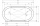On the 400 m athletic oval, two athletes started back to back from the same level. After the starting shot, they both ran away from each other at the same time. Athlete A ran at 15 km/h, and Athlete B ran at 17 km/h. A) in how many seconds did the athlete
• Runner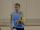Peter ran a speed of 12.4 km / h. After 45 minutes running he had a hiatus. The track is 31 km long. How many kilometers he still to run if he still running at the same speed?
• Scouts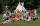The boys from scout group traveled 5 days distance 115 km. Every day walked 1.5 km less than the previous day. How many kilometers scouts walked in the first day?
• The hikerThe hiker will travel 40% of the route on the first day 1and/3 of the rest od second day. Last day 30 km. What was the length of the 3-day trip? How many kilometers did he walk each day?
• TruckThe truck passed 4550 km in 5 days. The first three days passed every day the same way. The fourth day passed 630 km and the fifth day was 920 km. How many km has passed the first three days?
• Car and motorcyclistA car and a motorcyclist rode against each other from a distance of 190 km. The car drove 10km/h higher than the motorcyclist and started half an hour later. It met a motorcyclist in an hour and thirty minutes. Determine their speeds.
• Journey 5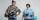A man has to do a journey of 84km in 3 hours. He travels the first 30km at 20km/hr. At what rate must he travel the remaining distance to complete his journey on time?
• Two runners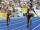Two runners ran simultaneously towards each other from locations distant 34.6 km. The average speed of the first runner was 1/5 higher than the average speed of the second runner. How long should each ran a 34.6 km, if you know that they meet after 67 min
• The cyclistThe cyclist drove from A to B, 60 km away. The first half drove at 30km/h, the second at 20km/h. How long did it take for him?
• Train speed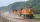The train speed is decreased during 50 sec from 72 km/h to 36 km/h. Assuming that the train movement is equally slowing, find the the acceleration and the distance that it travels at.
• The touristThe tourist traveled 78km in 3 hours. Part of the journey went on foot at 6km/h, the rest of the trip by bus at 30km/h. How long did he walk?
• Pedestrian up-down hill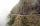Pedestrian goes for a walk first at the plane at 4 km/h, then uphill 3 km/h. Then it is in the middle of the route, turns back, and goes downhill at speed 6 km/h. The total walk was 6 hours. How many kilometers went pedestrian?
• Cross-country competition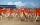Cross-country competition was attended by 76% of pupils of class 9.B. Class consis of 16 boys, two of whom did not run and 4 girls did not run. How many girls go to class 9.B?
• Velocipedes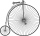In the 19th century, bicycles did not have a chain drive, and the pedals were connected directly to the wheel axis. This wheel diameter gradually increased until the so-called high bicycles (velocipedes) with a front wheel diameter of up to 1.5 meters, wh
• Accelerated motion - mechanicsThe delivery truck with a total weight of 3.6 t accelerates from 76km/h to 130km/h in the 0.286 km long way. How much was the force needed to achieve this acceleration?
• Sebastian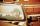From Kovalov Sebastian walks at 6 km / h started at 8:00 in the direction of Kuty. From Kuty, the godfather is driving at 50 km/h and started at 8:30. The distance is 24 km. When and where grandfather will take Sebastian to the car.